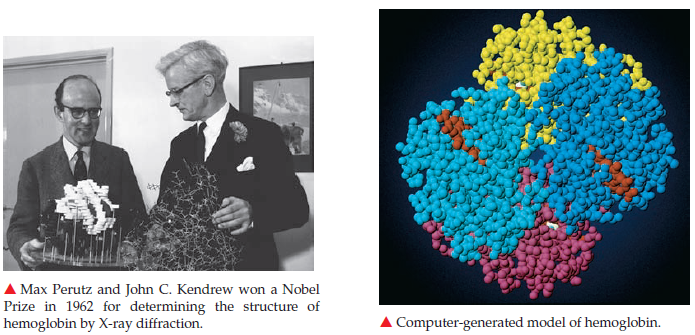×
Get Full Access to Introductory Chemistry - 5 Edition - Chapter 5 - Problem 106p
Get Full Access to Introductory Chemistry - 5 Edition - Chapter 5 - Problem 106p

×

# Solved: Molecules can be as small as two atoms or as large as thousands of atoms. InISBN: 9780321910295 34

## Solution for problem 106P Chapter 5

Introductory Chemistry | 5th Edition

• Textbook Solutions
• 2901 Step-by-step solutions solved by professors and subject experts
• Get 24/7 help from StudySoup virtual teaching assistantsIntroductory Chemistry | 5th Edition

4 5 1 352 Reviews
11
0
Problem 106P

Molecules can be as small as two atoms or as large as thousands of atoms. In 1962, Max F. Perutz and John C. Kendrew were awarded the Nobel Prize for their discovery of the structure of hemoglobin, a very large molecule that transports oxygen from the lungs to cells through the bloodstream. The chemical formula of hemoglobin is $$\mathrm{C}_{2852} \mathrm{H}_{4864} \mathrm{O}_{832} \mathrm{~N}_{812} \mathrm{~S}_{8} \mathrm{Fe}_{4}$$. Calculate the formula mass of hemoglobin.Equation Transcription:

C2952H4664O832N812S8Fe4.

Text Transcription:

C_2952H_4664O_832N_812S8F_e4.

Step-by-Step Solution:
Step 1 of 3

Solution 106P

Here, we have to calculate the formula mass of hemoglobin.

Formula mass of a compound can be calculated by using the following equation.

Formula mass = (no of atoms of the element ) x (atomic mass of 1st element) + (no of atoms of the element ) x (atomic mass of 2nd  element) +...

The chemical formula of Hemoglobin is  C2952H4664O832N812S8Fe4.

Formula mass of Hemoglobin =

2952 x (atomic mass of C- atoms) + 4664 x (atomic mass of H- atom ) +832 x (atomic mass of O atoms) + 812 x (atomic mass of N atoms) + 8 x (atomic mass of S atoms) + 4 x(atomic mass of Fe-atoms)

= 2952 x 12.01 amu + 4664 x 1.008 amu + 832 x 16 amu + 812 x 14.0 amu + 8 x 32.065 amu + 4 x 55.845amu

= 35453.52 amu +4701.312 amu + 13312 amu + 11368 amu +256.52 amu + 223.38 amu

= 65314.732 amu

Thus, the  formula mass of Hemoglobin is 65314.732 amu.

Step 2 of 3

Step 3 of 3

##### ISBN: 9780321910295

This full solution covers the following key subjects: Hemoglobin, max, prize, perutz, nobel. This expansive textbook survival guide covers 19 chapters, and 2046 solutions. Introductory Chemistry was written by and is associated to the ISBN: 9780321910295. This textbook survival guide was created for the textbook: Introductory Chemistry, edition: 5. Since the solution to 106P from 5 chapter was answered, more than 655 students have viewed the full step-by-step answer. The answer to “?Molecules can be as small as two atoms or as large as thousands of atoms. In 1962, Max F. Perutz and John C. Kendrew were awarded the Nobel Prize for their discovery of the structure of hemoglobin, a very large molecule that transports oxygen from the lungs to cells through the bloodstream. The chemical formula of hemoglobin is $$\mathrm{C}_{2852} \mathrm{H}_{4864} \mathrm{O}_{832} \mathrm{~N}_{812} \mathrm{~S}_{8} \mathrm{Fe}_{4}$$. Calculate the formula mass of hemoglobin. Equation Transcription:C2952H4664O832N812S8Fe4.Text Transcription:C_2952H_4664O_832N_812S8F_e4.” is broken down into a number of easy to follow steps, and 73 words. The full step-by-step solution to problem: 106P from chapter: 5 was answered by , our top Chemistry solution expert on 05/06/17, 06:45PM.

## Discover and learn what students are asking

Statistics: Informed Decisions Using Data : Applications of the Normal Distribution
?In Problems 19–22, find the value of ?? ?0.01

Statistics: Informed Decisions Using Data : Inference on the Least-Squares Regression Model and Multiple Regression
?State the requirements to perform inference on a simple least-squares regression line

Unlock Textbook Solution